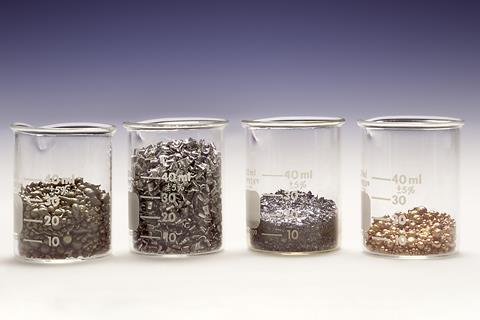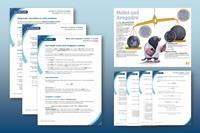Source: © Charles D Winters/Science Photo Library

What’s in a mole? Show your students the variation in a mole with this example: one mole of tin, magnesium, iodine and copper (left to right)

Whether making plastics, medicines or preparing materials for a chemical reaction, we have to measure the amounts we need. Atoms react in fixed proportions represented by the stoichiometry – the ratio of moles in a balanced equation for the reactants and products – of the reaction. Before conducting a reaction, we need to measure the number of these reactants. But, counting atoms is impossible, so we use the concept of the mole, which allows us to count atoms by measuring their mass.

One atom of helium has a relative atomic mass number of four (two protons and two neutrons), so it is four times as heavy as one atom of hydrogen (one proton). It follows that four grams of helium and one gram of hydrogen have the same number of atoms. Similarly, there is the same number of atoms in 12 grams of carbon. In fact, the relative atomic mass of all elements will have the same number of atoms. Experiments show that the number is 6.02214076 × 1023, known as Avogadro’s constant. We call one lot of this number one mole.

## What students need to know

The mole is the SI unit for the amount of substance. One mole contains exactly 6.02214076 x 1023 elementary entities. For students to fully understand the mole we need to expand beyond this core definition using class discussion to understand how to use a few specific definitions. Definitions to discuss and explore with students include:

1. A mole is a number consisting of exactly 6.02 x 1023 elementary entities.
2. The mole (symbol: mol) is the unit for the amount of substance.
3. The relationship between the number of moles, molar mass and mass is represented by this equation: number of moles (mol) = (mass (g))/(molar mass (g mol-1))
4. Historically, a mole was described as the number of atoms in exactly 12 g of carbon-12. However, on 20 May 2019 the General Conference on Weights and Measures set a mole as exactly 6.02214076 × 1023, as recommended by IUPAC, replacing the classic definition.

If you are new to teaching this topic at post-16, or need a refresher, the RSC on-demand CPD course on quantitative chemistry has sections devoted to the mole.

If you are new to teaching this topic at post-16, or need a refresher, the RSC on-demand CPD course on quantitative chemistry (rsc.li/3yyUvz8) has sections devoted to the mole.

## Common misconceptions

The mole is a difficult idea for students to grasp as a number because Avogadro’s constant is so large that they can’t imagine it meaningfully. Common student misconceptions include:

• mole refers only to molecules.
• mole is a certain mass (rather than a number).
• different substances of the same mass have the same number of particles.

So, at all stages, reaffirm that the mole is a number. Questioning is the best way to probe students’ understanding and discover misconceptions early before they’re embedded. BEST resources from STEM learning have a great selection of student worksheets on the mole that use diagnostic questions and tasks to identify and resolve misconceptions for pre-16 students. You can use these to provide a solid base to build on and unpick any embedded misconceptions.

It’s a tricky topic, but wonderful to see it click with students

Use examples and comparisons to ensure students understand, develop, question and test the concept of a mole. For example, directly address the question of the difference between one mole of hydrogen atoms and molecules. You can explore misconceptions in more depth and a possible teaching route by looking at this CPD article on moles and titrations.

Use examples and comparisons to ensure students understand, develop, question and test the concept of a mole. For example, directly address the question of the difference between one mole of hydrogen atoms and molecules. You can explore misconceptions in more depth and a possible teaching route by looking at this CPD article on moles and titrations: rsc.li/3ZHETVQ.

Visualising moles can help students link ideas. Providing physical examples helps them link the idea that all have the same number of elementary entities; this can be counted by measuring mass, and the coefficients of a reaction link to the proportions of moles. To help my students, I have a box of moles that contains the following in sealed containers:

• 12 g of carbon
• Two lots of 32.1 g of sulfur
• 55.8 g of iron
• 63.5 g of copper
• 24.3 g of magnesium
• 18 g of water
• 100.1 g of calcium carbonate
• 65.4 g of zinc
• 58.5 g of sodium chloride
• 87.9 g of iron sulfide

I can use my box of moles to physically represent one mole, as well as how mass and number are connected. I try to keep these in familiar forms where possible – iron as iron nails and magnesium as magnesium ribbon.

Analogies can be helpful when students are struggling with the concept of the mole. A common analogy is coins at a bank. A bank clerk doesn’t count pennies to calculate their total value. Instead, they measure the mass of the coins to count the number present. In the same way as with moles, the clerk needs to know the mass of each coin (molar mass, M) and the total mass of coins (mass, m) before using the same relationship we use to count atoms. Different denominations (1p, 2p, 5p, etc) have a different mass for each coin, so the relationship between mass and number varies depending on the mass of each coin (molar mass, M).

You could also use the analogy of bags of rice or cups. Or consider a resource that summarises the mole and Avogadro with the idea that one mole of moles has a mass only a little less than the moon.

•Moles infographic and resources, for age range 16–18

Use the poster, fact sheet and worksheet to get your students to apply their mathematical skills to three different problems involving moles.

You could also use the analogy of bags of rice or cups. Or consider a resource that summarises the mole and Avogadro with the idea that one mole of moles has a mass only a little less than the moon (rsc.li/3mOSTP2).

Moles infographic and resources, for age range 16–18

Get your students to apply their mathematical skills to three different problems involving moles.

Download the poster, fact sheet and worksheet from the Education in Chemistry website: rsc.li/3GvyR32

## Checking for understanding

Questioning is important to identify misconceptions before they become embedded. Use a combination of flashcards or a fact sheet to embed the meaning of the mole alongside other definitions. Then, follow up with practice questions and calculations.

Once students grasp the mole, regular retrieval and interleaving is essential to ensure they hold on to this understanding. For example, develop their understanding by asking them to determine the relative atomic mass of magnesium experimentally, and you can follow up with questioning to check their understanding.

## Take-home points

• It is impossible to count individual atoms, so we count them by measuring their mass.
• We use Avogadro’s constant to represent one mole.
• The molar mass has the same numerical value as the relative atomic mass, but in g mol-1.
• Students need to develop a conceptual understanding of the mole and how it relates to number, mass and proportions. It’s a tricky topic, but wonderful to see it click with students.

William Barron de Burgh is a chemistry teacher at Dame Elizabeth Cadbury School